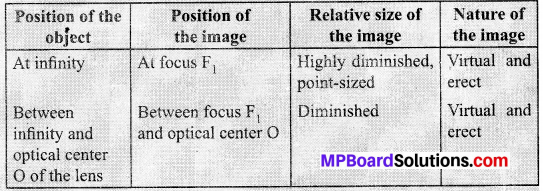## MP Board Class 10th Science Solutions Chapter 10 Light Reflection and Refraction

### MP Board Class 10th Science Chapter 10 Intext Questions

Class 10th Science Chapter 10 Intext Questions Page No. 168

Question 1.
Define the principal focus of a concave mirror.
The number of rays parallel to the principal axis are falling on a concave mirror which meat at a point is called principal focus of the concave mirror.

(or)

Light rays that are parallel to the principal axis of a concave mirror converge at a specific point on its principal axis after reflecting from the mirror. This point is known as the principal focus of concave mirror.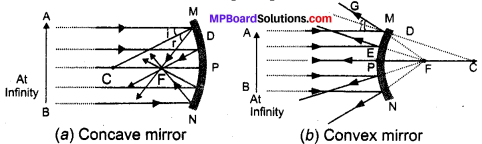Question 2.
The radius of curvature of a spherical mirror is 20 cm. What is its focal length?
R = 2f Here R = 20 cm
20 = 2f
∴ $$f=\frac { 20 }{ 2 } =10$$
∴ Focal length = 10 cm.

Question 3.
Name a mirror that can give an erect and enlarged image of an object.
Concave mirror can give an erect and enlarged image of an object when object is placed between the pole and principal focus.

Question 4.
Why do we prefer a convex mirror as a rear-view mirror in vehicles?
A convex mirror when fitted at rear-view position of vehicles, it gives a wider field of view, with which driver can see most of the traffic behind him. Convex mirrors give a virtual, erect and diminished image of the objects in front of it. So, we prefer a convex mirror as a rear-view mirror in vehicles.Class 10th Science Chapter 10 Intext Questions Page No. 171

Question 1.
Find the focal length of a convex mirror whose radius of curvature is 32 cm.
Radius of curvature, R = 32 cm
$$R=2f=\frac { R }{ 2 } =\frac { 32 }{ 2 } =16$$
∴ Convex mirror focal length is = 16cm

Question 2.
A concave mirror produces three times magnified (enlarged) real image of an object placed at 10 cm in front of it. Where is the image located?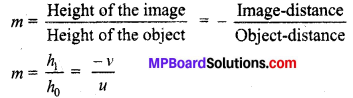Let the height of the object = h0 = h
Then, height of the image, h1 = -3h (Image formed is real)
= $$\frac { -3h }{ h }$$ = $$\frac { -v }{ u }$$
Object-distance, u = – 10 cm
v = 3 × (- 10)
= – 30 cm
Here, the negative sign indicates that an inverted image is formed at a distance of 30 cm in front of the given concave mirror.

Class 10th Science Chapter 10 Intext Questions Page No. 176

Question 1.
A ray of light travelling in air enters obliquely into water. Does the light ray bend towards the normal or away from the normal? Why?
Lightray bend towards normal. Because when a ray of light enters from rearer medium to denser medium, it changes its direction in the second medium.

Question 2.
Light enters from air to glass having refractive index 1.50. What is the speed of light in the glass? The speed of light in vacuum is 3 × 108 ms-1.
Refractive index of a medium:
µm = Speed of light in vacuum/Speed of light in the medium
Speed of light in vacuum, c = 3 × 108 ms-1
Refractive index of glass, µg = 1.50
Speed of light in the glass,
v = Speed of light in vacuum / Refractive index of glass
= c/µg
= 3 × 108/1.50
= 2 × 108 ms-1.

Question 3.
Find out, from Table the medium having highest optical density. Also find the medium w ith lowest optical density.
Table: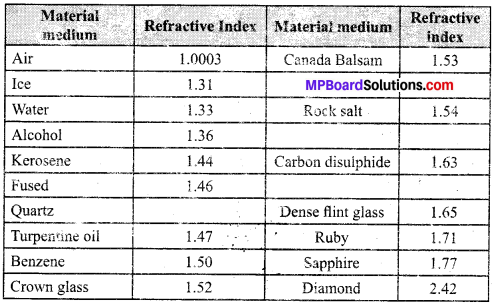Highest optical density = Diamond.
Lowest optical density = Air.

Optical density of a medium is proportional to the refractive index. Hence, medium with highest refractive index will have the highest optical density and vice-versa. It can be observed from table that diamond and air respectively have the highest and lowest refractive index. Therefore, diamond has the highest optical density and air has the lowest optical density.

Question 4.
You are given kerosene, turpentine and water. In which of these does the light travel fastest? Use the information given in table.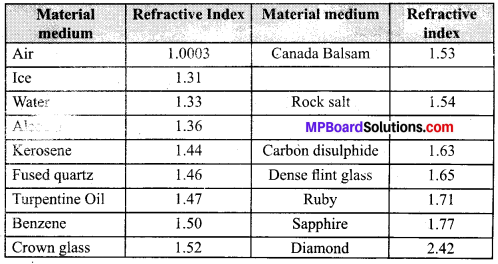Light travel faster in water when compared to kerosene and turpentine, since the refractive index of water is lower than kerosene and turpentine. The speed of light is inversely proportional to the refractive index.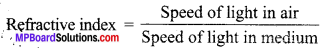Question 5.
The refractive index of diamond is 2.42. What is the meaning of this statement?
It means Ratio of velocity of light in air and velocity of air in diamond is 2.42.Class 10th Science Chapter 10 Intext Questions Page No. 184

Question 1.
Define 1 dioptre of power of a lens.
1 dioptre is the power of lens whose focal length is 1 metre 1 D = 1 m-1

Question 2.
A convex lens forms a real and inverted image of a needle at a distance of 50 cm from it. Where is the needle placed in front of the convex lens if the image is equal to the size of the object? Also, find the power of the lens.
Image of Needle is real and inverted means this is real image it is 2f
Image is at a distance of 50 cm
Hence needle is kept 50 cm in front of convex lens.
Distance of object, u = – 50 cm.
Distance of image v = 50 cm
Focal length f = ?
As per lens formula.f = 25 cm = 0.25 m
Power of the lensPower of the lens P = + 4D.

Question 3.
Find the power of a concave lens of focal length 2 m.
Focal length of concave lens, f = 2 m
Power of lens, P = $$\frac { 1 }{ f }$$ = $$\frac { 1 }{ (-2) }$$ = -0.5D### MP Board Class 10th Science Chapter 10 NCERT Textbook Exercises

Question 1.
Which one of the following materials cannot be used to make a lens?
(a) Water
(b) Glass
(c) Plastic
(d) Clay
(d) A lens allows light to pass through it, but clay does not have that property.

Question 2.
The image formed by a concave mirror is observed to be virtual, erect and larger than the object. Where should be the position of the object?
(a) Between the principal focus and the centre of curvature
(b) At the centre of curvature
(c) Beyond the centre of curvature
(d) Between the pole of the mirror and its principal focus.
(d) Between the pole of the mirror and its principal focus.

Question 3.
Where should an object be placed in front of a convex lens to get a real image of the size of the object?
(a) At the principal focus of the lens
(b) At twice the focal length
(c) At infinity
(d) Between the optical centre of the lens and its principal focus.
(b) When an object is placed at the centre of curvature in front of a convex lens, its image is formed at the centre of curvature on the other side of the lens.

Question 4.
A spherical mirror and a thin spherical lens have each a focal length of -15 cm. The mirror and the lens are likely to be:
(а) both concave
(b) both convex
(c) the mirror is concave and the lens is convex
(d) the mirror is convex, but the lens is concave
(а) both concave

Question 5.
No matter how far you stand from a mirror, your image appears erpct. The mirror is likely to be
(a) plane
(b) concave
(c) convex
(d) either plane or convex
(d) either plane or convex

Question 6.
Which of the following lenses would you prefer to use while reading small letters found in a dictionary?
(a) A convex lens of focal length 50 cm
(b) A concave lens of focal length 50 cm
(c) A convex lens of focal length 5 cm
(d) A concave lens of focal length 5 cm
(c)

Question 7.
We wish to obtain an erect image of an object, using a concave mirror of focal length 15 cm. What should be the range of distance of the object from the mirror? What is the nature of the image? Is the image larger or smaller than the object? Draw a ray diagram to show the image formation in this case.
Range of the distance of the object = 0 cm to 15 cm.
Nature of the image = virtual, erect and larger than the object.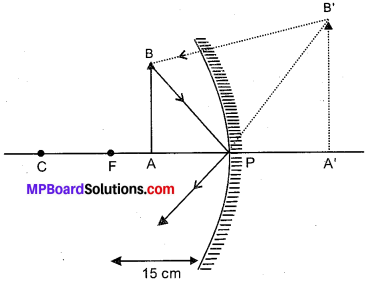Question 8.
Name the type of mirror used in the following situations.
(b) Side/rear-view mirror of a vehicle.
(c) Solar furnace.
(a) Concave mirror: Concave mirrors can produce powerful parallel ’ beam of light when the light source is placed at their principal focus. Hence, we can visualize ways easily in little light.

(b) Convex mirror: A convex mirror when fitted at rear view position of vehicles, it gives a wider field of view, with which driver can see most of the traffic behind him.

(c) Concave mirror: They are converging mirrors. This is because it concentrates the parallel rays of sun at principal focus and increase intensity of light falling on it.

Question 9.
One-half of a convex lens is covered with a black paper. Will this lens produce a complete image of the object? Verify your answer experimentally. Explain your observations.
Yes, the convex lens will form complete image of the object, even if its one half is covered with black paper. Following two cases can better explain it:

Case I : When the upper half of the lens is covered.
In this case, a ray of light coming from the object is being refracted by the lower half of the lens. These rays meet at the other side of the lens to form the image of the given object.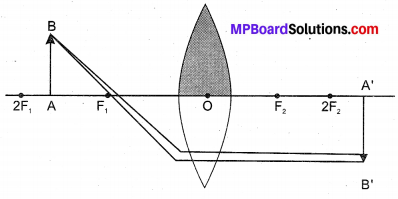Case II : If the lower half of the lens is covered.
In this case, a ray of light coming from the object is being refracted by the upper half of the lens. These rays meet at the other side of the lens to form the image of the given object.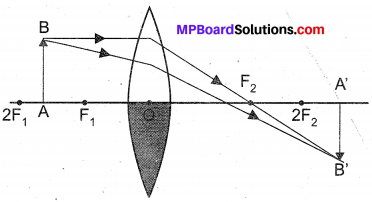Question 10.
An object 5 cm in length.is held 25 cm away from a converging lens of focal length 10 cm. Draw the ray diagram and find the position, size and the nature of the image formed.
Converging lens means a convex lens. As the distances given in the question are large, so we choose a scale of 1 : 5, i.e., 1 cm represents 5 cm. Therefore, on this scale 5 cm high object, object distance of 25 cm and focal length of 10 cm can be represented by 1 cm high, 5 cm and 2 cm lines respectively. Now, we draw the ray diagram as follows;

1. Draw a horizontal line to represent ‘die principal axis of the convex lens.
2. Centre line is shown by DE.
3. Mark two foci F and F’ on two sides of the lens, each at a distance of 2 cm from the lens.
4. Draw an arrow AB of height 1 cm on the left side of lens at a distance of 5 cm from the lens.
5. Draw a line AD parallel to principal axis and then allow it to pass straight through the focus (F’) on the right side of the lens.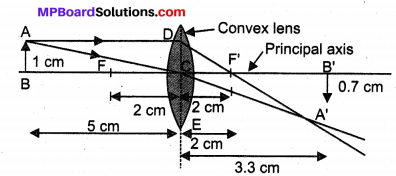6. Draw a line from A to C (centre of the lens), which goes straight without deviation.
7. Let the two lines starting from A meet at A’.
8. Draw A’B’, perpendicular to the principal axis from A’.
9. Now A’B represents the real, but inverted image of the object AB.
10. Then, measure CB’ and A’B’. It is found that CB’ = 3.3 cm and A’B’ = 0.7 cm.
11. Thus the final position, nature and size of the image A’B’ are
• (a) Position of image A’B’ = 3.3 cm × 5 = 16.5 cm from the lens on opposite side.
• (b) Nature of image A’B’: Real and inverted.
• (c) Height of image A’B’: 0.7 × 5 = 3.5 cm, i.e., image is smaller than the object.

Question 11.
A concave lens of focal length 15 cm forms an image 10 cm from the lens. How far is the object placed from the lens? Draw the ray diagram.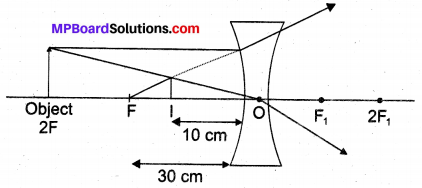Focal length of concave lens f = – 15 cm
Image distance, v = – 10 cm
According to the lens formula,
$$\frac { 1 }{ v }$$ – $$\frac { 1 }{ u }$$ = $$\frac { 1 }{ f }$$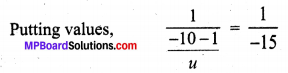On solving we get, u = – 30 cm
The negative value of u indicates that the object is placed 30 cm in front of the lens. This is shown in the above ray diagram.

Question 12.
An object is placed at a distance of 10 cm from a convex mirror of focal length 15 cm. Find the position and nature of the image.
Focal length of convex mirror,
f = +15 cm
Object distance, u = -10 cm
As per lens formulaMagnification $$=\frac{v}{u}=\frac{-6}{-10}=0.6$$
Virtual image is formed at the distance of 6 cm and it is erect.

Question 13.
The magnification produced by a plane mirror is +1. What does this mean?
The positive sign means image formed by a plane mirror is virtual and erect. Since, the magnification is I, it means that the size of the image is equal to the size of the object.

Question 14.
An object 5.0 cm in length is placed at a distance of 20 cm in front of a convex mirror of radius of curvature 30 cm. Find the position of the image, its nature and size.
Object distance, u = – 20 cm
Object height, h = 5 cm
Radius of curvature, R = 30 cm
Radius of curvature = 2 × focal length
R = 2f ⇒ f = 15 cm
According to the mirror formula,
$$\frac { 1 }{ v }$$ + $$\frac { 1 }{ u }$$ = $$\frac { 1 }{ f }$$
The positive value indicates that the image is formed behind the mirror.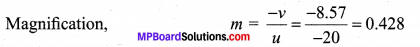The positive value of image height indicates image is virtual, erect and smaller in size.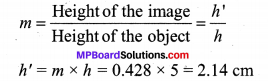Question 15
An object of size 7.0 cm is placed at 27 cm in front of a concave mirror of focal length 18 cm. At what distance from the mirror should a screen be placed, so that a sharp focussed image can be obtained? Find the size and the nature of the image.
Object-distance, u = – 27 cm
Object-height, h = 7 cm
Focal length, f = – 18 cm
According to the mirror formula,
$$\frac { 1 }{ v }$$ + $$\frac { 1 }{ u }$$ = $$\frac { 1 }{ f }$$
Putting values, $$\frac { 1 }{ v }$$ + $$\frac { 1 }{ (-27) }$$ = $$\frac { 1 }{ (-18) }$$
So, v = – 54 cm
The screen should be placed at a distance of 54 cm in front of the given mirror
and $$\frac{h_{2}}{h_{1}}=\frac{-v}{u}$$
h2 = -14 cm
The negative value of image indicates that the image is inverted.

Question 16.
Find the focal length of a lens of power – 2.0 D. What type of lens is this?
Given, P = -2D
Power of lens. p = $$\frac { 1 }{ f }$$
and, f = – $$\frac { 1 }{ 2 }$$
$$\frac{h_{2}}{h_{1}}=\frac{-v}{u}$$
= – 0.5 m
A concave lens, because it has a negative value of focal length.

Question 17.
A doctor has prescribed a corrective lens of power + 1.5 D. Find the focal length of the lens. Is the prescribed lens diverging or converging?
Given, P = 1.5.D
Power of lens, P = $$\frac { 1 }{ f }$$
and, focal length f = $$\frac { 1 }{ 1.5 }$$
= $$\frac { 10 }{ 15 }$$ = 0.66 m
A convex lens, because it has a positive focal length. Lens is converging.### MP Board Class 10th Science Chapter 10 Additional Important Questions

MP Board Class 10th Science Chapter 10 Multiple Choice Questions

Question 1.
The image formed by a convex lens is virtual, erect and larger than the object. The position of the object must be:
(a) Between the lens and its focus
(b) At the focus
(c) At twice the focal length
(d) At infinity
(a) Between the lens and its focus

Question 2.
A real image formed by a convex lens is always:
(a) On the same side of the lens as the object
(b) Erect
(c) Inverted
(d) Smaller than the object
(c) Inverted

Question 3.
If an object is moved towards a convex lens, the size of its image:
(a) Decreases
(b) Increases
(c) First decreases and then increases
(d) Remains the same
Ans.
(b) Increases

Question 4.
An object is placed at a distance of 30 cm from a concave mirror of focal length 15 cm. The image will be:
(a) Real and of same size
(b) Real and magnified
(c) Real and diminished
(d) virtual and magnified
(a) Real and of same size

Question 5.
A concave mirror always forms real and inverted image except when the object is placed:
(a) At infinity
(b) Between F and C
(c) At F
(d) Between F and pole of the mirror
(b) Between F and C

Question 6.
The mirror which has a wide field of view must be:
(a) Concave
(b) Convex
(c) Plane
(d) None of these
Ans.
(b) Convex

Question 7.
The image formed by a concave mirror:
(a) Is always real
(b) Is always virtual
(c) Can be both real and virtual
(d) None of these
Ans.
(c) Can be both real and virtual

Question 8.
An object is placed 20 cm from a convex lens of focal length 10 cm. The image must be:
(a) Real and diminished
(b) Real and of same size
(c) Real and enlarged
(d) Virtual and enlarged
(b) Real and of same size

Question 9.
The ratio of the focal length of spherical mirror to its radius of curvature is:
(a) 0.5
(b) 1
(c) 2
(d) 3
(a) 0.5

Question 10.
A real and inverted image of the same size is formed by a concave mirror when the object is placed:
(a) Between the mirror and its focus.
(b) Between the focus and the centre of curvature.
(c) At the centre of curvature.
(d) Beyond the centre of curvature.
Ans.
(c) At the centre of curvature.MP Board Class 10th Science Chapter 10 Very Short Answer Type Questions

Question 1.
What is a mirror? Mention the different types of mirrors commonly used.
Mirror: A highly polished surface which is smooth enough to reflect a good fraction of light incident on it is called a mirror. The mirror may be a highly polished metal surface or an ordinary glass plate coated with a thin silver layer.

Question 2.
What is the number of images of an object held between two plane parallel mirrors?
Infinity.

Question 3.
Does the refractive index for a given pair of media depend on the angle of incidence?
No, it is independent of the angle of incidence.

Question 4.
The refractive index of water with respect to air is $$\frac { 4 }{ 3 }$$. What is the refractive index of air with respect to water?
Refractive index of air with respect to water = $$\frac { 3 }{ 4 }$$

Question 5.
Can absolute refractive index of a medium exceed unity?
No, because speed of light is maximum in vacuum.

Question 6.
Why does a ray of light bend when it travels from one medium to another?
The bending of light or refraction occurs due to the change in the speed of light as it passes from one medium to another due to change in the density of the medium.

Question 7.
What happens when a ray of light strikes the surface of separation between the two media at right angle?
Ans.
The ray of light passes undeflected from one medium to another.
Here, ∠i = ∠r = 0°

Question 8.
What do you mean by a magnification less than unity?
It means that the size of the image is smaller than the size of the object.

Question 9.
Which spherical mirror has

1. a real focus and
2. a virtual focus?

1. A concave mirror has a real focus.
2. A convex mirror has a virtual focus.

Question 10.
State the position of the object for which a concave mirror produces virtual magnified image.
The object should be placed between F and P of the concave mirror.

MP Board Class 10th Science Chapter 10 Short Answer Type Questions

Question 1.
Name the type of mirror(s) that should be used to obtain:
(i) a magnified and virtual image.
(ii) a diminished and virtual image of an object.
Draw labelled diagrams to show the formation of the required image in each of the above two cases. Which of these mirrors could also form a magnified and real image of the object? State the position of object for which this could happen.
(i) Concave mirror.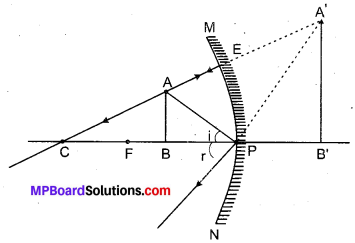Fig. 10.3 : Concave mirror with the object between F and P.

(ii) Convex mirror.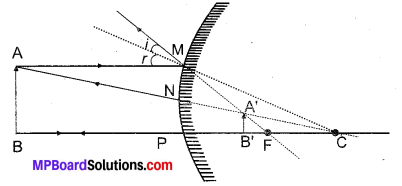Fig. 10.4: Convex mirror with the object between pole and infinity.

Question 2.
Explain the uses of concave and convex mirrors.
Uses of concave mirrors:
1. Shaving mirror : A concave mirror is used as a shaving or make-up mirror because it forms erect and enlarged image of the face when it is held closer to the face.

2. As head mirror : E.N.T. specialists use a concave mirror on their forehead. The light from a lamp after reflection from the mirror is focussed into the throat, ear or nose of the patient making the affected part more visible.

3. In ophthalmoscope : It consists of a concave mirror with a small hole at its centre. The doctor looks through the hole from behind the mirror while a beam of light from a lamp reflected from it, is directed into the pupil of patient’s eye which makes the retina visible.

4. In headlights : Concave mirrors are used as reflectors in headlights of motor vehicles railway engines, torch lights etc. The source is placed at the focus of the concave mirror. The light rays after reflection travel over a. large distance as a parallel beam of high intensity.

5. In astronomical telescopes : A concave mirror of large diameter (5 m or more) is used as objective in an astronomical telescope. It collects light from the sky and makes visible even those faint stars which cannot be seen with naked eye.

6. In solar furnaces : Large concave mirrors are used to concentrate sunlight to produce heat in solar furnace.

Uses of convex mirrors : Drivers use convex mirror as a rear-view mirror in automobiles because of the following two reasons:

1. A convex mirror always forms an erect, virtual and diminished image of an object placed anywhere in front of it.
2. A convex mirror has a wider field of view than a plane mirror of the same size as shown in Fig. 10.5.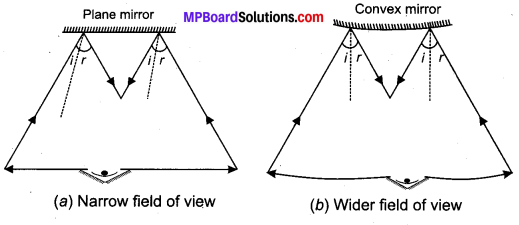Fig. 10.5: Field of view of (a) a plane mirror, (b) a convex mirror.

Thus, convex mirrors enable the driver to view much larger traffic behind him than would be possible with a plane mirror. The main disadvantage of a convex mirror is that it does not give the correct distance and the speed of the vehicle approaching from behind.

Question 3.
State the characteristics of the image formed by a convex mirror. What is the value of angle of incidence and angle of reflection when a ray of light retraces its path after reflection from a convex mirror? Illustrate with the help of a ray diagram.
Properties of the image formed by a convex mirror:

(a) The image is always virtual and erect.
(b) The image is highly diminished or point sized.
(c) It is always formed between F and P.
(d) As the object is moved towards the pole of a convex mirror, image also moves towards its pole and gradually increases in size till its size becomes almost equal to that of the object.

When array of light retraces its path, ∠i = ∠r = 0°.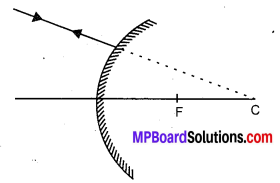Fig. 10.6: A ray directed towards C is reflected back along same path after reflection from a convex mirror.

Question 4.
State the new Cartesian sign convention followed for reflection of light by spherical mirrors.
According to this convention:

1. The object is on the left of the mirror. So all the ray diagrams are drawn with the incident light travelling from left to right.
2. All the distances parallel to the principal axis are measured from the pole of the mirror.
3. All distances measured in the direction of incident light are taken as positive.
4. All distances measured in the opposite direction of incident light are taken as negative.
5. Heights measured upwards and perpendicular to the principal axis are taken positive.
6. Heights measured downwards and perpendicular to the principal axis are taken negative.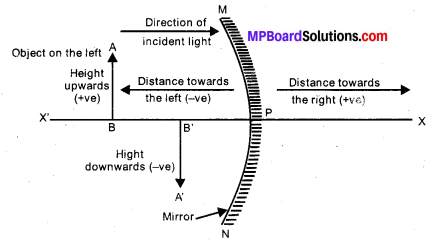Fig. 10.7: New Cartesian sign convention for reflection of light by spherical mirrors.

Question 5.
State the type of mirror preferred as
(i) rear view mirror in vehicles
(ii) shaving mirror. Justify your answer giving two reasons in each case.
(i) A convex mirror is preferred as a rear-view mirror because:

(a) It always forms an erect, virtual and diminished image of an object placed anywhere in front of it.
(b) It has wider field of view.

(ii) A concave mirror is preferred as a shaving mirror because when it is held closer to the face, it forms:

(a) an enlarged image of the face.
(b) an erect image of the face.

Question 6.
State the laws of refraction of light.
Laws of refraction of light: The refraction of light obeys the following two laws:
1st law: The incident ray, the refracted ray and normal to the interface of two transparent media at the point of incidence, all lie in the same plane.

2nd law: The ratio of the sine of the angle of incidence to the sine of the angle of refraction is constant for a given pair of media.
Mathematically,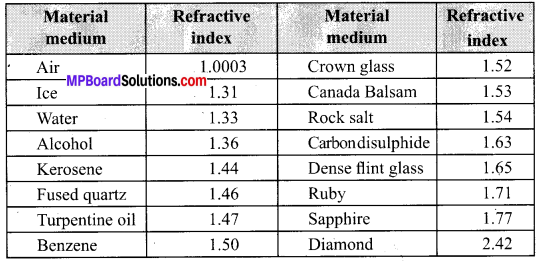The ratio µ21 is called refractive index of the second medium with respect to the first medium. The second law of refraction is also called Snell’s law of refraction.

Question 7.
What is the physical significance of refractive index?
The refractive index of any medium gives the ratio of the speed of light in vacuum to the speed of light in that medium. For example, the refractive index of water, µw = 1.33. This means that the ratio of the speed of light in vacuum or air to the speed of light in water is 1.33.

Question 8.
What do you mean by optically denser and optically rarer media? How is the speed of light related to optical density?
The optical density of a medium represents its ability to refract light. A medium having larger refractive index is called optically denser medium than the other. The other medium having lower refractive index is called optically rarer medium.

The speed of light is higher in a rarer medium than a denser medium. Thus, a ray of light travelling from a rarer medium to a denser medium slows down and bends towards the normal.

When it travels from a denser medium to a rarer medium, it speeds up and bends away from the normal.

Table:
Refractive indices of some material media (with respect to vacuum)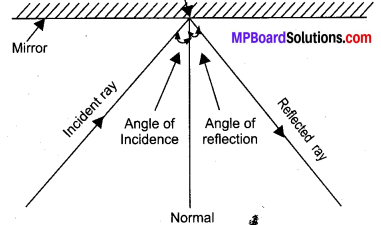MP Board Class 10th Science Chapter 10 Long Answer Type Questions

Question 1.
With the help of a ray diagram, state and explain the laws of reflection of light at a plane mirror. Mark the angles of incidence and reflection clearly on the diagram.
As shown in Fig. 10.8, when a ray of light is incident on a mirror, it gets reflected in accordance with the following laws of reflection.
1st law: The incident ray, the reflected ray and the normal at the point of incidence all lie in the same plane.
2nd law: The angle of incidence (i) is equal to the angle of reflection (r) i.e.∠i = ∠r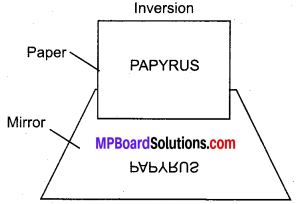Fig.10.8: Reflection in a plane mirror.

Question 2.
What is lateral inversion of an image? What is the cause of lateral inversion?
Lateral inversion: If we stand before a plane mirror and move our right hand, our image appears to move its left hand. In fact, our entire image is reversed sideways. This sideways reversal of the image is known as lateral inversion.

Cause of lateral inversion: Lateral inversion is due to the fact that in a plane mirror the image is as far behind the mirror as the object is in front of it, and that the front of the image and the front of the object face each other. The laterally inverted image of the word PAPYRUS is as shown in Fig. 10.9. The images of symmetrical letters like A, H, I, M, O, T, U, V, W, X, Y, 8 are not affected by lateral inversion.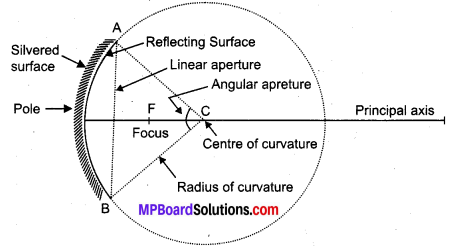Fig.10.9: Lateral inversion before a mirror.

Question 3.
Define the following terms in connection with spherical mirrors:
(i) Angular aperture
(ii) Centre of curvature
(iv) Principal axis
(v) Linear aperture
(vi) Pole
(vii) Principal force
(viii) Focal length
(ix) Principle focus Focal plane.
Definition in connection with spherical mirrors: In Fig 10.10, let APB be a principal section of a spherical mirror, i.e., the section cut by a plane passing through pole and centre of curvature of the mirror
(i) Angular aperture : It is the angle ACB subtended by the boundary of the spherical mirror at its centre of curvature.
(ii) Centre of curvature : It is the centre C of the sphere of which the mirror forms a part.
(iii) Radius of curvature : It is the radius R (= AC or BC) of the sphere of which the mirror forms a part.
(iv) Principal axis : The line passing through the pole and the centre of curvature of mirror is called its principal axis.
(v) Linear aperture : It is the diameter AB of the circular boundary of the spherical mirror.
(vi) Pole: It is the middle point P of the spherical mirror.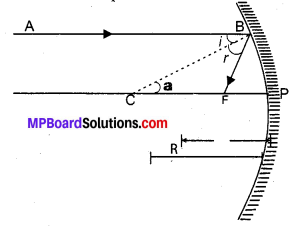Fig. 10.10: Characteristics of a concave mirror.

Question 4.
Deduce a relation between focal length (f) and radius of curvature (R) for a concave mirror.
Relation between f and R for a concave mirror: As shown in Fig. 10.11, consider a ray AB parallel to the principal axis and incident at the point B of a concave mirror. After reflection from the mirror, this ray passes through its focus F, obeying the laws of reflection. If C is the centre of curvature, then CP = R, is the radius of curvature and CB is normal to the mirror at point B.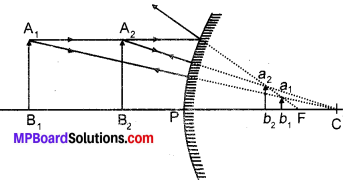Fig. 10.11: Relation betweenTand R for a concave mirror.

According to the law of reflection, ∠i = ∠r
As AB is parallel to CP, so ∠a = ∠i (Alternate angles)
∠a = ∠r
Thus, ∆ BCF is isosceles,
Hence, CF = FB.
If the aperture (or size) of the mirror is small, then B lies close to P, so that,
FB = FP
FP = CF = $$\frac { 1 }{ 2 }$$ CP
or f = $$\frac { R }{ 2 }$$
or Focal lenght = $$\frac { 1 }{ 2 }$$ × Radius of curvature
Thus, the principal focus of a spherical mirror lies midway between the pole and the centre of curvature.

Question 5.
What happens to the size of the image formed by a convex mirror, when an object is gradually moved towards the mirror?
When the object is at position A1B1, its virtual image is at a1b1 When the object is at position A2B2, its virtual image is at a2b2. So, when an object is gradually moved towards the pole of a convex mirror, its image also moves towards its pole and gradually increases in size till it has a size almost equal to that of the object. However, the image is always formed between F and P.### MP Board Class 10th Science Chapter 10 NCERT Text Book Activities

Class 10 Science Activity 10.1 Page No. 161

• Take a large shining spoon. Try to view your face in its curved surface.
• Do you get the image? Is it smaller or larger?
• Move the spoon slowly away from your face. Observe the image. How docs it change?
• Reverse the spoon and repeat the Activity. How does the image look like now?
• Compare the characteristics of the image on the tw o surfaces.

Observations:

• The image is formed that is smaller in size.
• On moving the spoon away, the size of the image gradually decreases with increasing field of view.
• On reversing the spoon, spoon w hen dose forms elect and magnified image on the inner curved ..urface. As we move away, image gets inverted and gradually decrease in Its size.
• The image on outer surface of spoon is erect and gradually decreases in size on moving away. The image of spoon on inner surface is erect and gets inverted on moving away. The size also decreases when moved away.

Class 10 Science Activity 10.2 Page No. 162

Caution: Do not look at the Sun directly or even into a mirror reflecting sunlight. It may damage your eyes.

• Hold a concave mirror in your hand and direct its reflecting surface towards the Sun.
• Direct the light reflected by the mirror on to a sheet of paper held close to the mirror.
• Move the sheet of paper back and forth gradually until you find on the paper sheet a bright, sharp spot of light.
• Hold the mirror and the paper in the same position for a few minutes. What do you observe? Why?

Observations:

• The paper starts burning when the mirror and paper are held in the same position for a few minutes as light rays from the sun, sharply focuses on this point and due to which heat concentrates at a point resulting in burning because of intense heating.

Class 10 Science Activity 10.3 Pages No. 163,164

You have already learnt a way of determining the focal length of a concave mirror. In activity 10.2, you have seen that the sharp bright spot of light you got on the paper is, in fact, the image of the Sun. It was a tiny, real, inverted image. You got the approximate focal length of the concave mirror by measuring the distance of the image from the mirror:

• Take a concave mirror. Find out its approximate focal length in the w ay described above. Note down the value of focal length. (You can also find it out by obtaining image of a distant object on a sheet of paper.)
• Mark a line on a table with a chalk. Place the concave mirror on a stand. Place the stand over the line such that its pole lies over the line.
• Draw with a chalk two more lines parallel to the previous line such that the distance between any two successive lines it equal to the focal length of the minor. These lines will now correspond to the positions of the points P, F and C, respectively. Remember – For a spherical mirror of small aperture, the principal focus F lies midway between the pole P and the centre of curvature C.
• Keep a bright object, say a burning candle, at a position far beyond C. Place a paper screen and move it in front of the mirror till you obtain a sharp bright image of the candle flame on it.
• Observe the image carefully. Note down its nature, position and relative size with respect to the object size.
• Repeat the activity by placing the candle-(a) just beyond C, (b) at C, (c) between F and C. (d) at F, and (c) between P and F.
• In one of the cases, you may not get the image on the screen. Identify the position of the object in such a case, Then, look for its virtual image in the mirror itself.
• Note down and tabulate your observations.

Observations: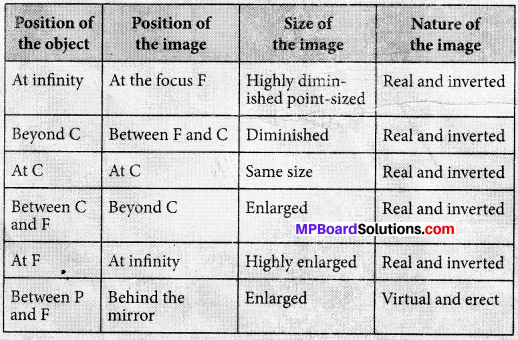Class 10 Science Activity 10.4 Page No. 166

• Draw neat ray diagrams for each position of the object shown in previous activity 10.3 observations.
• You may take any two of the rays mentioned in the previous section for locating the image.
• Compare your diagram with those given in Fig. 10.1.
• Describe the nature, position and relative size of the image formed in each case.
• Tabulate the results in a convenient format.

Observations: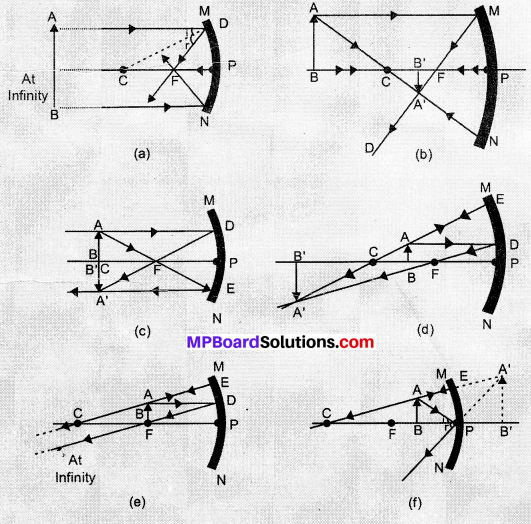Fig. 10.1: Ray diagrams for the image formation by a concave mirror.

 Position of the object Position of the. image Size of the image Nature of the image At infinity At the focus F, behind the mirror Highly diminished, point-sized Virtual and erect Between infinity and the pole P of the mirror Between P and F, behind the mirror Diminished Virtual and erect

Class 10 Science Activity 10.5 Page No. 167

• Take a convex mirror. Hold it in one hand.
• I Iold a pencil in the upright position in the other hand.
• Observe the image of the pencil in the mirror. Is the image erect or inverted? Is it diminished or enlarged?
• Move the pencil away from the mirror slowly. Does the image become smaller or larger?
• Repeat this Activity carefully. State whether the image will move closer to or farther away from the focus as the object is moved away from the min or?

Observations:

• The image is erect and diminished. The image becomes smaller or moving pencil away.
• The image moves closer to the focus as the object is moved away from the mirror.

Class 10 Science Activity 10.6 Page No 167

• Observe the image of a distant object, say a distant tree, in a plane mirror.
• Could you see a full-length image?
• Try with plane minors of different sizes. Did you see the entire object in the image?
• Repeat this Activity with a concave mirror. Did the mirror show full length image of the object?
• Now try using a convex mirror. Did you succeed? Explain your observations with reason.

Observations:

• No, full – length image of a distant object is not seen in a plane mirror.
• The entire images of the objects were not seen.
• No, the mirror do not show full length image of the object.
• Yes, with the convex mirror we can see full length image of distant object with under field of view’ this is because the convex mirror are used as rear or side view mirrors in vehicles. The image formed is diminished, erect and virtual.

Class 10 Science Activity 10.7 Page No. 172

• Place a coin at the bottom of a bucket filled with water.
• With your eye to a side above water, try to pick up the coin in one go. Did you succeed in picking up the coin?
• Repeat the Activity. Why did you not succeed in doing it in one go?

Observations:

• No, we cannot succeed in picking up the coin.
• This happens due to refraction, the coin appears to he at some other place from where it is actually present. The light rays coming out from water tends to bend creating this problem.

Class 10 Science Activity 10.8 Page No. 172

• Place a large shallow bowl on a Table and put a coin in it.
• Move away slowly from the bowl. Stop when the coin just disappears from your sight.
• Ask a friend to pour water gently into the bowl without disturbing the coin.
• Keep looking for the coin from your position. Does the coin becomes visible again from your position? How could this happen?

Observations:

• Yes, on pouring water, it again becomes visible and little raised due to refraction.

Class 10 Science Activity 10.9 Page No. 172

• Draw a thick straight line in ink, over a sheet of white paper placed on a Table.
• Place a glass slab over the line in such a way that one of its edges make an angle with the line.
• Look at the portion of the line under the slab from the sides. What do you observe? Does the line under the glass slab appear to be bent at the edges ?
• Next, place the glass slab such that it is normal to the line. What do you observe now’ Does the part of the line under the glass slab appear bent?
• Look at the line from the top of the glass slab. Does the part of the line, beneath the slab, appear to be raised? Why does this happen?

Observations:

• The line under the glass slab appear bent at the edges due to refraction.
• No, now it does not appear bend as a ray of light perpendicular to the plane of a refracting medium does not change angle during refraction.
• Yes, this is also due to refraction that apparent position of image of object seems nearer than its actual position.

Class 10 Science Activity 10.10 Page No. 173

• Fix a sheet of white paper on a drawing board using drawing pins.
• Place a rectangular glass slab over the sheet in the middle.
• Draw the outline of the slab with a pencil. Let us name the outline as ABCD.
• Take four identical pins.
• Fix two pins, say E and F, vertically such that the line joining the pins is inclined to the edge AB.
• Look for the images of the pins E and F through the opposite edge. Fix two other pins, say G and H, such that these pins and the images of E and F lie on a straight line.
• Remove the pins and the slab.
• Join the positions of tip of the pins E and F and produce the line up to AB. Let EF meet AB at O. Similarly, join the positions of tip of the pins G and H and produce it up to the edge CD. Let HG meet CD at O’.
• Join O and O’. Also produce EF up to P, as shown by a dotted line in Fig. 10.2.

Observations: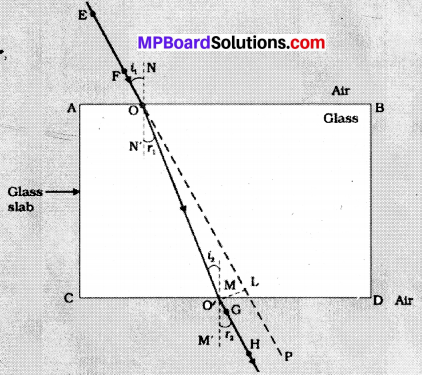Fig. 10.2: Refraction of light through a rectangular glass slab.

Class 10 Science Activity 10.11 Page No. 177

Caution: Do not look at the Sun directly or through a lens while doing this Activity or otherwise. You may damage your eyes if you do so.

• Hold a convex lens in your hand. Direct it towards the Sun.
• Focus the light from the Sun on a sheet of paper. Obtain a sharp bright image of the Sun.
• Hold the paper and the lens in the same position for a while. Keep observing the paper. What happened? Why? Recall your experience in Activity 10.2.

Observations:

• This is due to sharp focusing of all rays at a single point after passing through the lens. The concentration of light rays increases at a point resulting in burning of paper.

Class 10 Science Activity 10.12 Page No. 178

• Take a convex lens. Find its approximate focal length in a way described in Activity 10.11.
• Draw five parallel straight lines, using chalk, on a long Table such that the distance between the successive lines is equal to the focal length of the lens.
• Place the lens on a lens stand. Place it on the central line such that the optical centre of the lens lies just over the line.
• The two lines on cither side of the lens correspond to F and 2F of the lens respectively. Mark them with appropriate letters such as 2F1, F1, F2 and 2F2, respectively.
• Place a burning candle, far beyond 2F, to the left. Obtain a clear sharp image on a screen on the opposite side of the lens.
• Note down the nature, position and relative size of the image.
• Repeat this Activity by placing object just behind 2F1 between F1 and 2F1 at F1, between F1 and O. Note down and tabulate your observations.

Observations: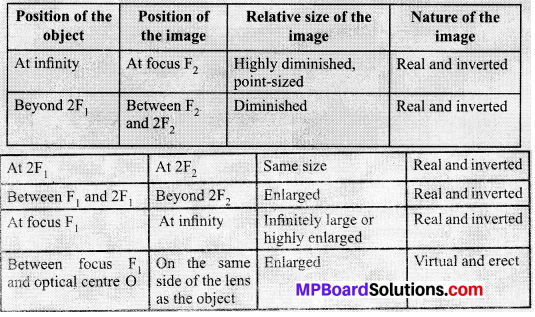Class 10 Science Activity 10.13 Page No. 179

• Take a concave lens. Place it on a lens stand.
• Place a burning candle on one side of the lens.
• Look through the lens from the other side and observe the image. Try to get the image on a screen, if possible. If not, observe the image directly through the lens.
• Note down the nature, relative size and approximate position of the image.
• Move the candle away from the lens. Note the change in the size of the image. What happens to the size of the image when the candle is placed too far away from the lens.

Observations: